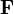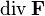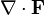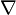# Divergence facts for kids

Kids Encyclopedia Facts

In mathematics, divergence is a differential operator that associates a vector field with a scalar field. In a vector field, each point of the field is associated with a vector; in a scalar field, each point of the field is associated with a scalar.

Given a vector field$\mathbf{F}$, the divergence of$\mathbf{F}$ can be written as$\operatorname{div} \mathbf{F}$ or$\nabla \cdot \mathbf{F}$, where$\nabla$ is the gradient and$\cdot$ is the dot product operation.

Divergence is used to formulate Maxwell's equations and the continuity equation.

## Related pagesDivergence Facts for Kids. Kiddle Encyclopedia.